Design of Experiments Guide > Accelerated Life Test Designs > Example of an Accelerated Life Test Design
Publication date: 11/10/2021

# Example of an Accelerated Life Test Design

Use the ALT designer to construct a failure probability optimal ALT test design. In this example, suppose that you need to design an accelerated life test for a mechanical component. The single acceleration factor is torque, and the normal use stress is 35 Nm (newton meters). You want to estimate the time at which 10% of the units fail at the normal use stress. You construct a failure probability optimal ALT test.

Your test plan has the following characteristics:

A total of 100 units are available for testing.

The life distribution is assumed to be Weibull.

The life-stress relationship is given by the logarithmic transformation.

You plan to test torque at three stress levels: 50, 75, and 100 Nm.

You have prior knowledge to predict initial failure times at the test levels. See Obtain Prior Estimates.

The test runs for 5,000 cycles.

You will monitor the process for failures on a continuous basis.

Obtain Prior Estimates

To create an accelerated life test design, you must provide prior estimates of the parameters. Here is an approach to obtaining prior estimates:

1. Use your process knowledge to create a table of hypothetical, but likely, failure times at a small number of stress levels.

2. Use the Fit Life by X platform to fit a model and obtain estimates of the model parameters.

3. Use these estimates as your prior values for creating a design using the ALT Design platform.

Following the approach outlined above, you create a data table containing estimates of the number of failure cycles for a balanced design. Your table consists of five units at each of the three stress levels to be used in your design. Your data table is Torque Prior.jmp, found in the Sample Data Library.

1. Select Help > Sample Data Library and open Design Experiment/Torque Prior.jmp.

2. Select Analyze > Reliability and Survival > Fit Life by X.

3. Select Cycles and click Y, Time to Event.

4. Select Torque and click X.

5. From the Relationship list, select Log.

6. Enter 35 for the Use Condition. Recall that 35 Nm is the normal use condition.

7. From the Distribution list, select Weibull.

Figure 22.2 Fit Life by X Launch Window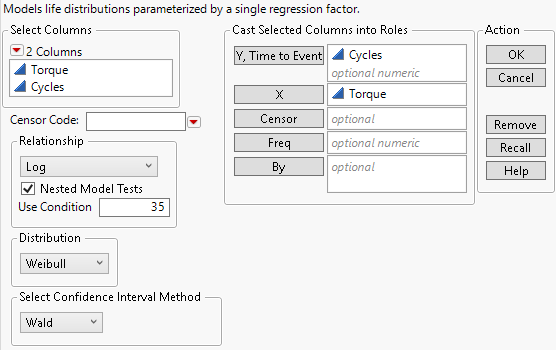8. Click OK.

9. Scroll down to the Weibull Results outline and open the Correlation Matrix outline.

Figure 22.3 Fit Life by X Model for Prior Data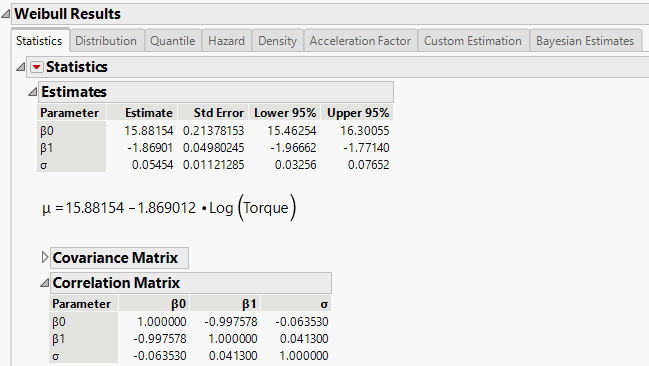The model for the mean is given in the Estimates outline. The Estimate column contains the parameter estimates for the intercept (β0), the linear coefficient (β1), and the scale (σ). The standard errors column contains the standard errors for the estimates. The estimated correlations for the parameter estimates are given in the Correlation Matrix outline. Use these parameter estimates, standard errors, and correlations as your prior values to construct your ALT design.

Enter Basic Specifications

To create an accelerated life test design define accelerating factors, use conditions, and test conditions.

1. Select DOE > Special Purpose > Accelerated Life Test Design.

Notice that One accelerating factor and Continuous Monitoring are selected by default.

2. Click Continue.

3. Under Factor Name, click X1 and type Torque.

Notice that the Number of Levels is set to 3 by default.

4. Select Log under Factor Transformation.

5. Enter 35 for both the Low Usage Condition and the High Usage Condition.

Setting both the low and high usage conditions to 35 indicates that 35 is the normal usage condition.

6. Enter 50 for the Low Test Condition and 100 for the High Test Condition.

Figure 22.4 Completed ALT Specification Window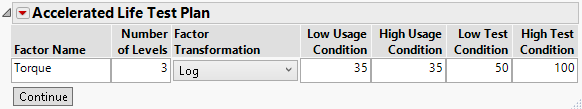7. Click Continue.

8. Notice the Torque Level Values are set to 50, 75, and 100.

Note: JMP sets the values of the levels evenly spaced between the low and high test conditions. You can change the levels.

9. Ensure that Weibull is selected as the Distribution Choice.

Enter Prior Information and Remaining Specifications

Continue the construction of the example ALT design by entering prior information.

1. Under Prior Mean, select Specify Intercept and enter the prior acceleration model parameter estimates that you obtained using the Fit Life by X platform with your hypothetical data:

Enter 15.88 for Intercept.

Enter -1.87 for Power (Torque).

Enter 0.05 for1/β (scale parameter for a Weibull model).

2. Select Specify prior uncertainty.

3. Enter the estimated standard errors and correlations from the prior acceleration model. Figure 22.3 shows the completed Prior Uncertainty outline:

Figure 22.5 Completed Prior Specification Outline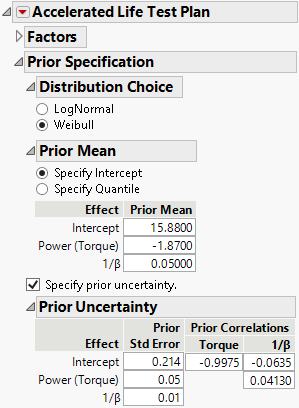Click the Accelerated Life Test Plan red triangle and select ALT Optimality Criterion > Make Failure Probability Optimal.

You plan to conduct the test over 5,000 cycles. You are interested in predictions for as many as 10,000 cycles.

4. In the Diagnostic Choices outline, enter 10,000 for both boxes for Time range of interest.

5. In the Design Choices outline. Enter 5,000 for Length of test and 100 for Number of units under test.

Figure 22.6 Completed Diagnostic and Design Choices Outline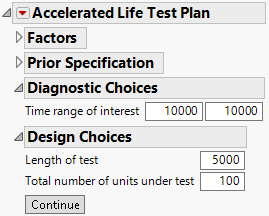Make the Design

Continue the ALT example by generating the design.

1. Click Continue.

Figure 22.7 Balanced Design Diagnostics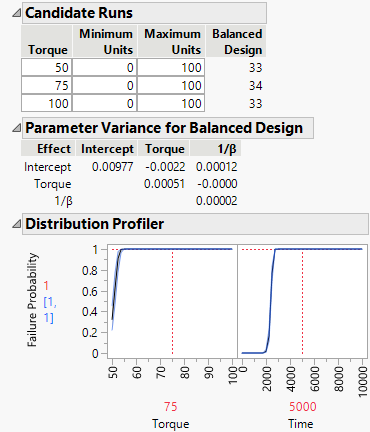The number of runs in a balanced design appears in the Candidate Runs outline. The Parameter Variance for Balanced Design outline shows the covariance matrix for the parameters for this design. The Distribution Profiler also appears. When you obtain the optimal design, you can compare the Parameter Variance and Distribution Profiler results to those for the balanced design to see the reduction in uncertainty.

2. Click Make Design.

The optimal experimental design appears, along with other results.

Figure 22.8 Optimal Design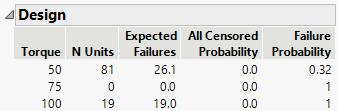The optimal design is computed based on the levels of the test runs, the total number of units to be tested, and the prior information that you specified. The optimal design consists of testing the specified number of units at each torque level:

81 units at 50 Nm

0 units at 75 Nm

19 units at 100 Nm

Run Simulations

Evaluate the example ALT design with the simulator. Use the simulator to simulate the results of your test plan based on the assumptions used to build the design. This is a way to evaluate if the test plan is sufficient before running the test plan.

1. Enter 100 for the Number of Simulations.

2. Enter 0.1 for the Simulation Probability of Interest.

3. Enter 35 for the Simulation Usage for Torque.

4. Click Run Simulations to run 100 simulations of the experiment. This is to check to see whether the 100 units that you plan to test will be sufficient given your prior assumptions for the design.

Figure 22.9 Simulation Results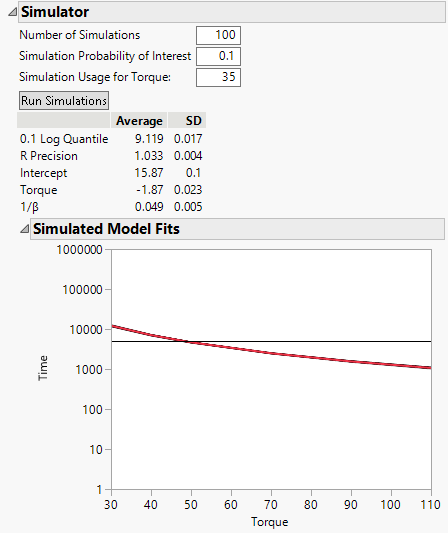The simulated model fits shows that the 100 simulations of the model are all similar as they all fall close to the average shown by the red curve. The Y axis is on a log scale.

5. Double-click the Y axis and select Linear from the Type menu and click OK.

The variability in the simulated fits around the 35 normal usage condition is now easier to view. You expect variability in your estimated of the normal usage condition. The simulation helps you evaluate if your ALT plan is acceptable. If there is too much variability, you might need to increase the number of units on test or adjust the test conditions.

Save Design

Finally, complete the Example ATL design by making a test plan and saving it to a data table.

1. Click Make Test Plan to save the design to a data table. This table is a summary of the design.

2. Click Make Table to save the design to a data table. This table has 100 rows, one for each test run.